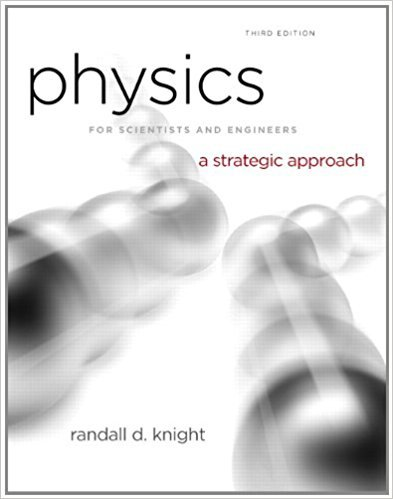×
Log in to StudySoup
Get Full Access to Physics For Scientists And Engineers: A Strategic Approach With Modern Physics - 3 Edition - Chapter 6 - Problem 2cq
Join StudySoup for FREE
Get Full Access to Physics For Scientists And Engineers: A Strategic Approach With Modern Physics - 3 Edition - Chapter 6 - Problem 2cq

Already have an account? Login here
×
Reset your password

# A ball tossed straight up has v = 0 at its highest point.ISBN: 9780321740908 69

## Solution for problem 2CQ Chapter 6

Physics for Scientists and Engineers: A Strategic Approach with Modern Physics | 3rd Edition

• Textbook Solutions
• 2901 Step-by-step solutions solved by professors and subject experts
• Get 24/7 help from StudySoup virtual teaching assistantsPhysics for Scientists and Engineers: A Strategic Approach with Modern Physics | 3rd Edition

4 5 1 400 Reviews
19
4
Problem 2CQ

Problem 2CQ

A ball tossed straight up has v = 0 at its highest point. Is it in equilibrium? Explain.

Step-by-Step Solution:

Solution 2CQ

Step 1 of 2:

No, The ball is not in equilibrium.

There are two reasons to explain. The first reason is given as follows. Even though the velocity of the ball is zero at the highest point, the ball is still acted by gravity. The acceleration is not zero here.

In the case of equilibrium, the net force acting on the object must be zero. But the ball is attracted by the gravity and doesn’t have zero net force.

Step 2 of 2

##### ISBN: 9780321740908

The answer to “A ball tossed straight up has v = 0 at its highest point. Is it in equilibrium? Explain.” is broken down into a number of easy to follow steps, and 18 words. Physics for Scientists and Engineers: A Strategic Approach with Modern Physics was written by and is associated to the ISBN: 9780321740908. This textbook survival guide was created for the textbook: Physics for Scientists and Engineers: A Strategic Approach with Modern Physics, edition: 3. The full step-by-step solution to problem: 2CQ from chapter: 6 was answered by , our top Physics solution expert on 08/30/17, 04:34AM. Since the solution to 2CQ from 6 chapter was answered, more than 264 students have viewed the full step-by-step answer. This full solution covers the following key subjects: ball, Equilibrium, explain, highest, its. This expansive textbook survival guide covers 17 chapters, and 1439 solutions.

Unlock Textbook Solution

Enter your email below to unlock your verified solution to:

A ball tossed straight up has v = 0 at its highest point.